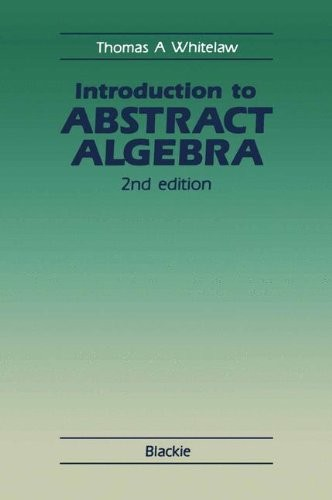# Introduction to Abstract Algebra by Thomas A. Whitelaw

In Stock
£11.99
N/A
Only 1 left

## Introduction to Abstract Algebra Summary

### Introduction to Abstract Algebra by Thomas A. Whitelaw

THIS IS A TEXTBOOK FOR STUDENTS UNDERTAKING, OR ABOUT TO UNDERTAKE, A first course in Abstract Algebra (e. g. second-year Honours students and second-or third-year Ordinary Degree students in Scottish universities; first- year students in many English universities). The first edition of the book proved helpful to many such students, and it is hoped that this second edition may be found even more helpful. The main changes made in producing the se co nd edition have been the inclusion of a whole new chapter on the symmetrie group and the expansion of the existing sets of exercises, together with updating and revising of the text. The book is not an advanced treatise on group theory or on any kindred part of mathematics. But, in contrast to many elementary textbooks, it does penetrate far enough into Abstract Algebra to let the student see that the subject has worthwhile insights to offer, and to introduce hirn to some of the distinctive ways of thinking that produce interesting results. For example, in group theory, the material covered includes cyclic groups, Lagrange's theorem, homomorphisms, normal subgroups, quotient groups, and (in the new chapter) the partition of the symmetrie group of degree n into conjugacy classes and an intro duc ti on to the alternating group of degree n. Although there is only one chapter on rings (and fields), it is a lengthy chapter and covers a wide variety of ideas.

### Why buy from World of BooksOur excellent value books literally don't cost the earthFree delivery in the UKEvery used book bought is one saved from landfill

One Sets and Logic.- 1. Some very general remarks.- 2. Introductory remarks on sets.- 3. Statements and conditions quantifiers.- 4. The implies sign (?).- 5. Proof by contradiction.- 6. Subsets.- 7. Unions and intersections.- 8. Cartesian product of sets.- Exercises.- Two Some Properties of ?.- 9. Introduction.- 10. The well-ordering principle.- 11. The division algorithm.- 12. Highest common factors and Euclid's algorithm.- 13. The fundamental theorem of arithmetic.- 14. Congruence modulo m(m??).- Exercises.- Three Equivalence Relations and Equivalence Classes.- 15. Relations in general.- 16. Equivalence relations.- 17. Equivalence classes.- 18. Congruence classes.- 19. Properties of ?m as an algebraic system.- Exercises.- Four Mappings.- 20. Introduction.- 21. The image of a subset of the domain; surjections.- 22. Injections; bijections; inverse of a bijection.- 23. Restriction of a mapping.- 24. Composition of mappings.- 25. Some further results and examples on mappings.- Exercises.- Five Semigroups.- 26. Introduction.- 27. Binary operations.- 28. Associativity and commutativity.- 29. Semigroups: definition and examples.- 30. Powers of an element in a semigroup.- 31. Identity elements and inverses.- 32. Subsemigroups.- Exercises.- Six an Introduction to Groups.- 33. The definition of a group.- 34. Examples of groups.- 35. Elementary consequences of the group axioms.- 36. Subgroups.- 37. Some important general examples of subgroups.- 38. Period of an element.- 39. Cyclic groups.- Exercises.- Seven Cosets and Lagrange's Theorem on Finite Groups.- 40. Introduction.- 41. Multiplication of subsets of a group.- 42. Another approach to cosets.- 43. Lagrange's theorem.- 44. Some consequences of Lagrange's theorem.- Exercises.- Eight Homomorphisms, Normal Subgroups, and Quotient Groups.- 45. Introduction.- 46. Isomorphic groups.- 47. Homomorphisms and their elementary properties.- 48. Conjugacy.- 49. Normal subgroups.- 50. Quotient groups.- 51. The quotient group G/Z.- 52. The first isomorphism theorem.- Exercises.- Nine the Symmetric Group Sn.- 53. Introduction.- 54. Cycles.- 55. Products of disjoint cycles.- 56. Periods of elements of Sn.- 57. Conjugacy in Sn.- 58. Arrangements of the objects 1, 2, n.- 59. The alternating character, and alternating groups.- 60. The simplicity of A5.- Exercises.- Ten Rings.- 61. Introduction.- 62. The definition of a ring and its elementary consequences.- 63. Special types of ring and ring elements.- 64. Subrings and subfields.- 65. Ring homomorphisms.- 66. Ideals.- 67. Principal ideals in a commutative ring with a one.- 68. Factor rings.- 69. Characteristic of an integral domain or field.- 70. Factorization in an integral domain.- 71. Construction of fields as factor rings.- 72. Polynomial rings over an integral domain.- 73. Some properties of F[X] where F is a field.- Exercises.- Appendix to Exercises.

GOR004816542
Introduction to Abstract Algebra by Thomas A. Whitelaw
Thomas A. Whitelaw
Used - Very Good
Paperback